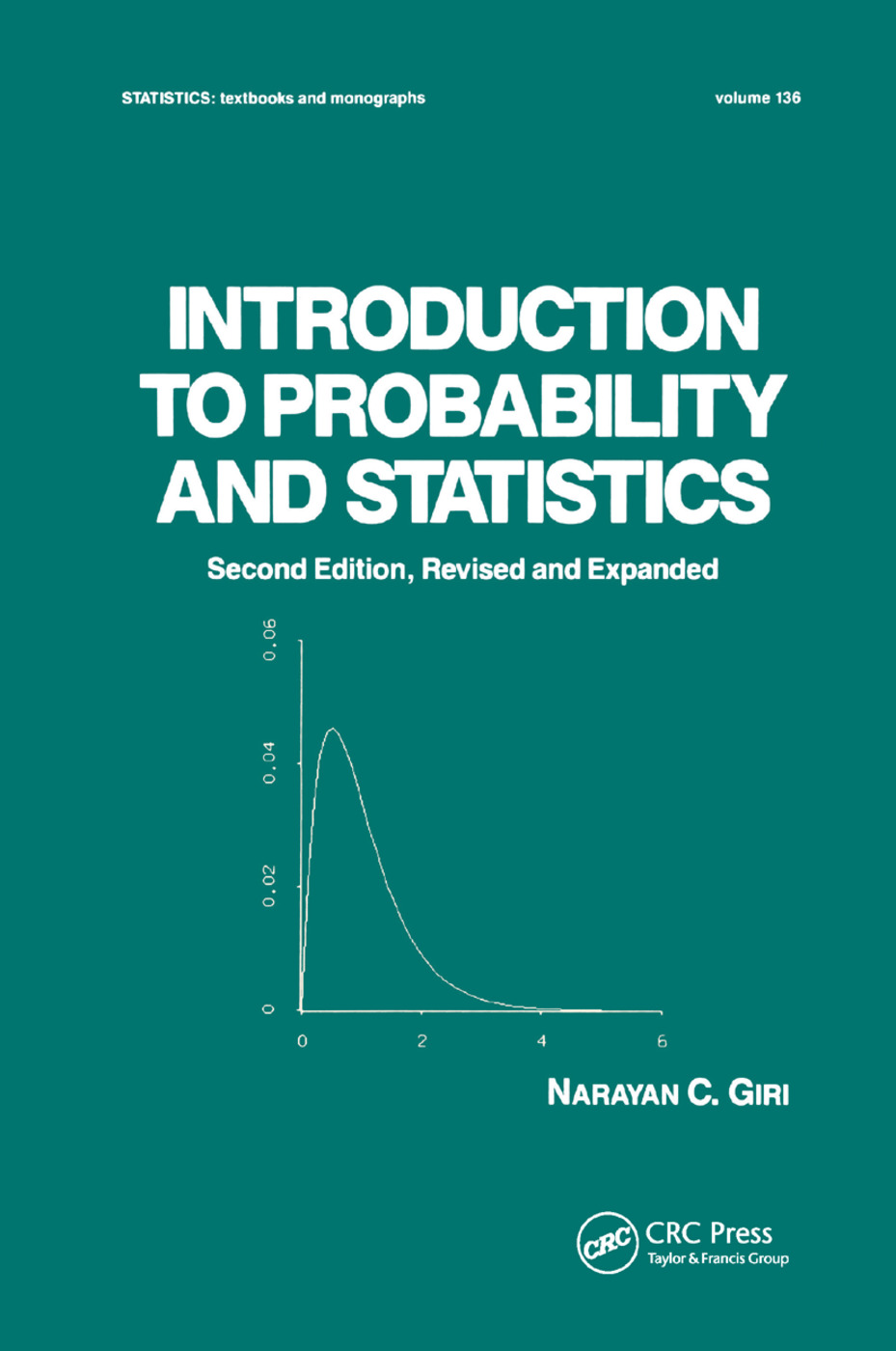# Introduction to Probability and Statistics

## 2nd Edition

CRC Press

560 pages

Paperback: 9780367402396
pub: 2019-06-19
\$74.95
x
Hardback: 9780824790370
pub: 1993-04-20
\$135.00
x
eBook (VitalSource) : 9780203749920
pub: 2019-01-22
from \$37.48

FREE Standard Shipping!

### Description

Beginning with the historical background of probability theory, this thoroughly revised text examines all important aspects of mathematical probability - including random variables, probability distributions, characteristic and generating functions, stochatic convergence, and limit theorems - and provides an introduction to various types of statistical problems, covering the broad range of statistical inference.;Requiring a prerequisite in calculus for complete understanding of the topics discussed, the Second Edition contains new material on: univariate distributions; multivariate distributions; large-sample methods; decision theory; and applications of ANOVA.;A primary text for a year-long undergraduate course in statistics (but easily adapted for a one-semester course in probability only), Introduction to Probability and Statistics is for undergraduate students in a wide range of disciplines-statistics, probability, mathematics, social science, economics, engineering, agriculture, biometry, and education.

Introduction; general concepts of probability; random variables, probability distributions, and characteristic functions, stochastic convergence and limit theorems; concepts of statistics; univariate distributions; multivariate distributions; order statistics and related distributions; statistical inference - parametric point estimation; testing of statistical hypotheses; large-sample methods; statistical decision theory; sequential analysis; nonparametric methods; general linear hypothesis and analysis of variance; some applications of analysis of variance; appendix A - vectors and matrices; appendix B - statistical tables.